# Graph, oriented

(diff) ← Older revision | Latest revision (diff) | Newer revision → (diff)

directed graph

A graph with an orientation assigned to each of its edges. An oriented graphis specified by a set of verticesand a setof ordered pairs of vertices, known as arcs. One says that the arc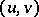issues from the vertexand enters the vertex. The number of arcs issuing fromis known as the output semi-degree of, while the number of arcs enteringis known as the input semi-degree of. An alternating sequence of vertices and arcs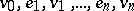in which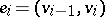,, is known as an (oriented) sequence. The sequence is closed if its first and last vertices coincide. A path is a sequence in which all vertices are different. A contour is a non-trivial closed sequence (containing at least one arc) in which all vertices, except the first and last ones, are different. If a path from a vertexto a vertexexists, then one says thatis reachable from.

An oriented graphwith numbered verticesand arcs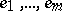can be specified by an incidence matrix, i.e. by the matrix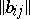of dimensionin which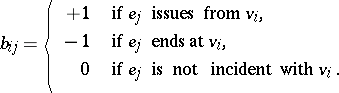The adjacency matrix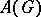of the vertices of an oriented graphis the matrixof dimension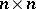in which the entry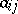is equal to the number of arcs going from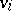to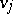. The sums of the elements in the rows ofare equal to the output semi-degrees of the vertices of the oriented graph, while the sums of the elements in the columns are equal to the input semi-degrees. An entry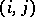of the matrix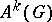(i.e. the-th power of the adjacency matrix of) is equal to the number of sequences of lengthgoing fromto.

Several types of connectivity can be defined in an oriented graph (cf. Graph, connectivity of a). An oriented graph is said to be strongly connected, or strong, if any two of its vertices are mutually reachable; it is unilaterally connected if, out of any two of its vertices, at least one is reachable from the other; it is weakly connected, or weak, if any two of its vertices are connected by a chain in the graph, such chain having been obtained from the initial oriented graph by replacing each arc by a (non-oriented) edge.

Oriented graphs are used in: probability theory for the representation of a Markov chain; the theory of games in the description of the set of game situations and results of competitions; mathematical economics when solving transportation problems; the theory of automata in constructing transition diagrams, etc. In graph theory itself, an orientation can be introduced in solving certain problems concerning non-oriented graphs, thus reducing the initial problem to a problem on oriented graphs. The basic difference between an oriented and a non-oriented graph is manifested in the definitions of concepts such as a path, connectivity, reachability, distance, etc. The most interesting types of oriented graphs are tournaments (cf. Tournament), transitive graphs, graphs of partial orders, growing trees, graphs of one-place mappings, and contour-free graphs.

How to Cite This Entry:
Graph, oriented. Encyclopedia of Mathematics. URL: http://encyclopediaofmath.org/index.php?title=Graph,_oriented&oldid=13648
This article was adapted from an original article by A.A. Sapozhenko (originator), which appeared in Encyclopedia of Mathematics - ISBN 1402006098. See original article